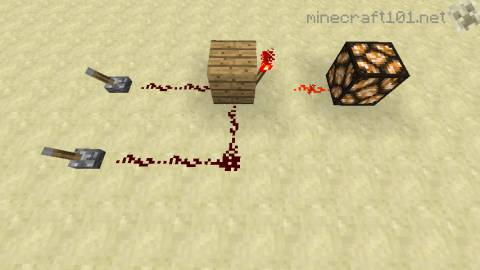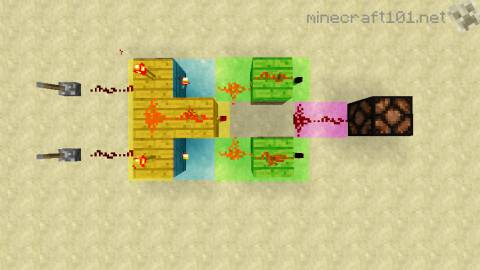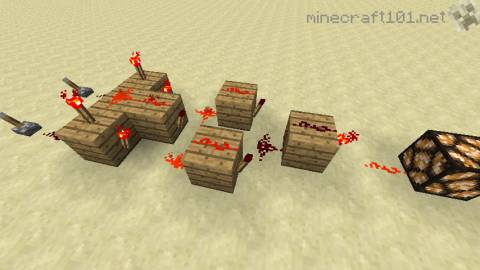# Redstone Logic

This is a very basic introduction to logic circuits in Minecraft. These are basic circuits that you can use to achieve simple tasks. It's possible to make this a lot more complicated, but we won't do that here! Here are the basics, presented as simply as possible.

You should read the 'redstone basics' introductory page first, if you haven't already.

## Logic gates

A logic gate is a circuit that is on or off depending on certain inputs. They can be very useful. For instance, you might want to control a lamp so that it only comes on when a daylight sensor is NOT giving a signal. Or you might want to open a door if a button is pushed OR someone is standing on a pressure plate. Those words in capitals, NOT and OR, are the names of simple logic gates.

The best way to learn these is to built them yourself and see what happens when you flip the levers. In all cases, its input on the left, and the output is a redstone lamp on the right.

### NOT gateThis is the simplest gate: it's a redstone torch attached to a block. If the block is powered, the torch is off; otherwise the torch is on. In other words, the output is opposite to the input. This gate is also sometimes called an inverter.

### OR gateThis is so simple it almost doesn't count! Two possible power sources are connected to this lamp: if either one, or both, is on, then the lamp will be on.In practice, you will want to include repeaters in your circuits so that the current only goes in the right direction.

### NOR gate

The NOR gate gives an output if neither of the inputs is on; it's the opposite of an OR gate.The Minecraft version is made by wiring up two inputs to a NOT gate. It's that easy!

### AND gateThis gate only gives an output if both inputs are on. How does it work? There are two torches on top: each one is switched off by one of the inputs. The torch that is connected to the output will only come if both of those torches are switched off.

### NAND gate

NAND is short for "Not AND", and it gives the opposite output to an AND gate. It will give an output if either or neither input is on, but not if both are.In construction, you can see that this is similar to the AND gate: (in fact, the AND gate is actually a combination of a NAND plus a NOT).

### XOR gate

XOR stands for 'eXclusive OR', and it's a gate that gives an output if one, but only one, of the inputs is on. There are many ways to build this, but this is probably the easiest:

How does it work?The yellow part is an AND gate.

Each of the blue parts is a NOT gate, one for each input. These invert the signal from the input.

Each of the green parts is a NOR gate that will only come on if: (a) the yellow AND gate is off and (b) the inverted signal from the input is off (i.e. the input is on).

The pinkish part is just a simple OR gate that collects the signal from either side of the gate.

### XNOR gate

This is the opposite of the XOR gate, and it's made by simply sticking a NOT gate on the end of a XOR.